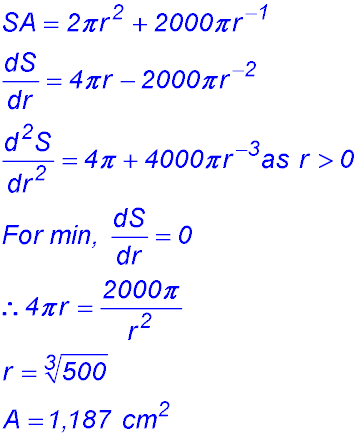Dr. J's Maths.com
Where the techniques of Maths
are explained in simple terms.

Calculus - Differentiation - Applied max/min questions.
Type 3: Basic 3D shapes - Test Yourself 1 - Solutions.

 Boxes 1. (i)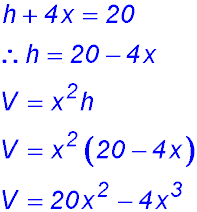(ii)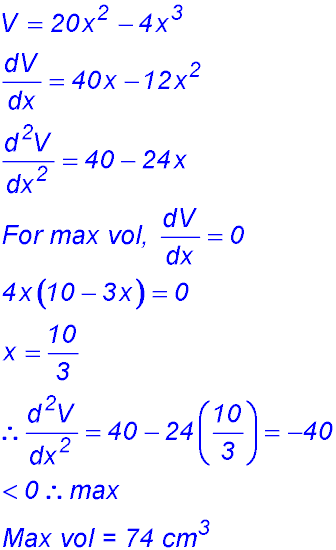2. (i) The three horizontal pieces are each 2x × y cm2 in area. The two ends are each xy cm2 in area and the back panel is 2x×x cm2. Hence the use of the pineboard is 24 = 3(2xy) + 2(xy) + 2x2 = 8xy + 2x2. (ii) Volume = x × 2x × y = 2x2y. But from part (i)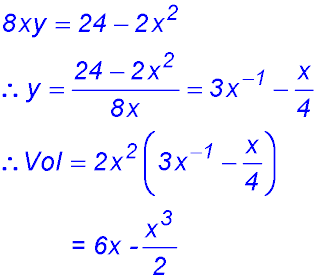(iii)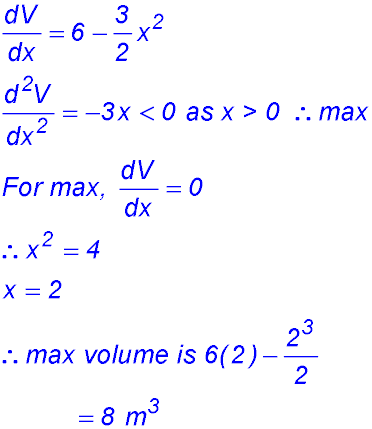3. (i)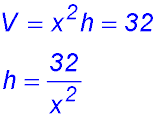(ii)4. (i) To find the area of the trapezium cross section, we need the width at the top. This width has a central length equal to the base "a" plus two lengths from isosceles triangles note the angle given is 45°). So those lengths are both h. Top = a + h + h = a + 2h = 60.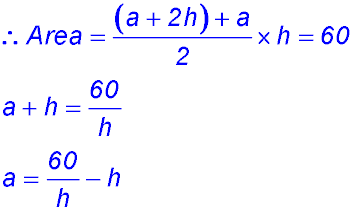(ii)(iii)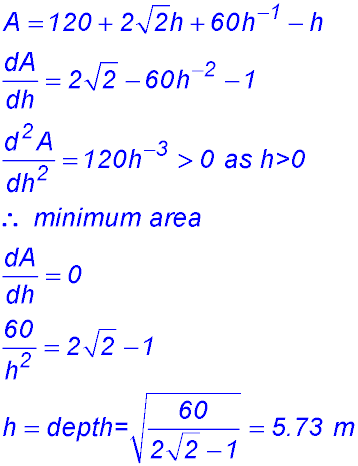5. (i)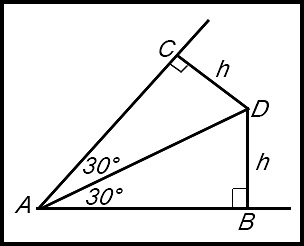Take the lower left corner of the shape and note the mark-up in the diagram above.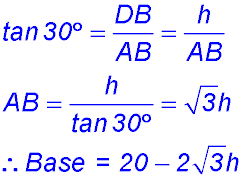(ii)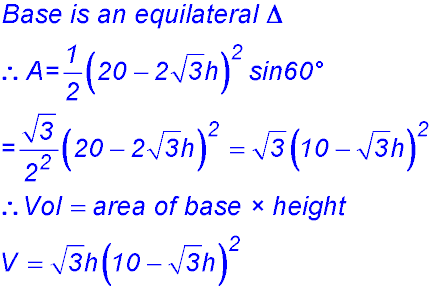(iii)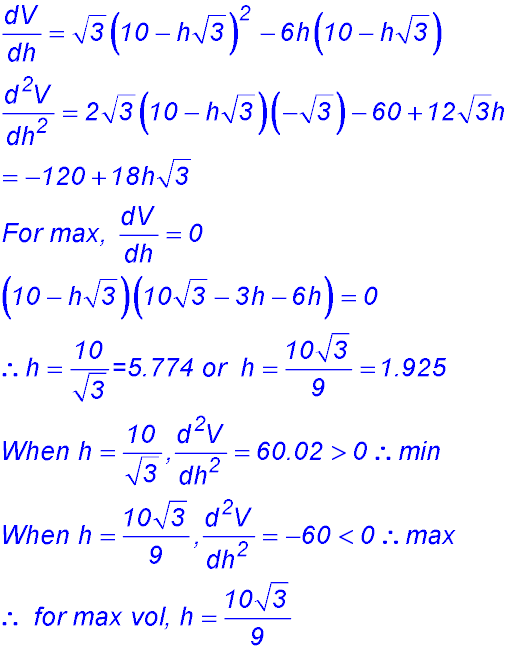Cones. 6.7.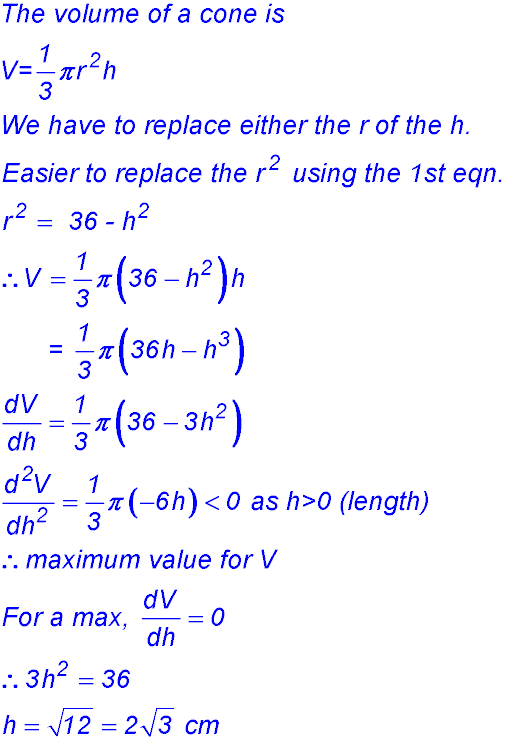8. (i)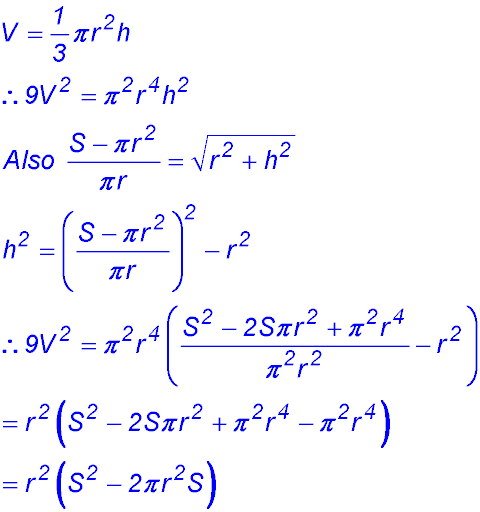(ii)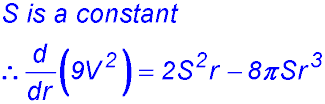(iii) 9. Cylinders. 10.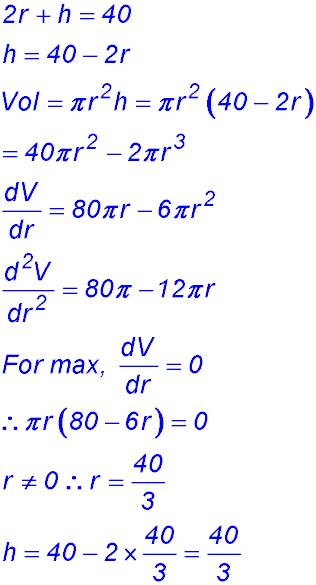11.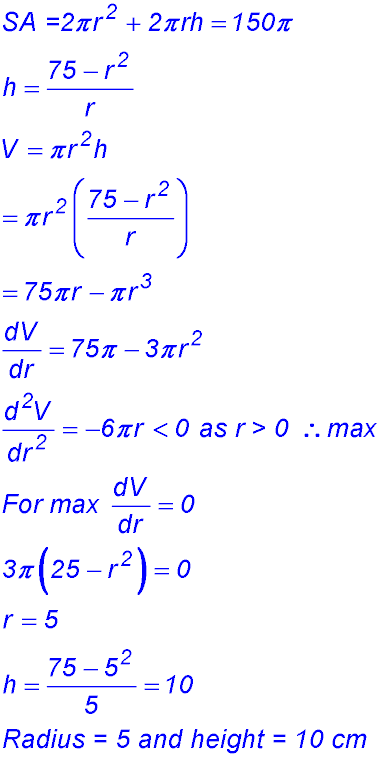12. (i)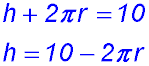(ii)(iii)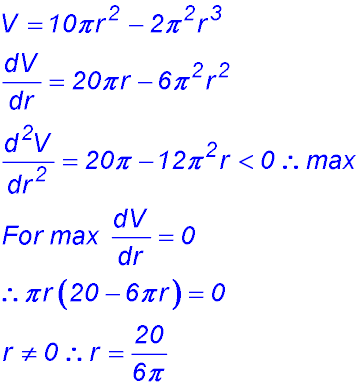(iv)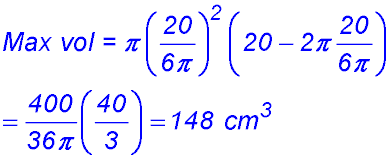13.14. (i)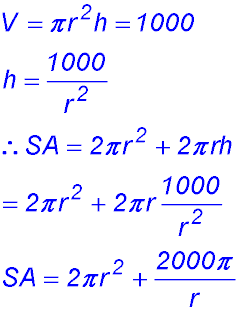(ii)